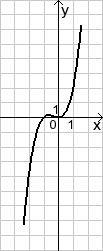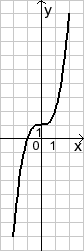# Match Equations to Curves

In this worksheet, students match equations to the given curve.Key stage:  KS 4

Curriculum topic:  Algebra

Difficulty level:### QUESTION 1 of 10

This worksheet is about matching curves to equations.

Example

Match the curve with its equation:y = x3 −2      OR    y = x3 +x2      OR     y = x2 - 2

Answer

Look at the curve.  It has a cubic shape so will definitely have an x3 in the equation.

Then look at what happens when x = 0.

It passes through (0,0), so the equation cannot be y = x3 −2

The equation is  y = x3 +x2

(check that this passes through (-1, 0). When x = -1, y = -1 + 1 = 0

Choose the correct equation for the given curve:y = x3 −2

 y = 1 + 3 x

y = x3 + x2

Choose the correct equation for the given curve:y = 1 + 3 x

y = x2 −2

 y = 1 + 1 x

Choose the correct equation for the given curve:y = x3 −2

y = x2 −2

y = x3 −3

Choose the correct equation for the given curve:y = x3 + 4x2

y = x2 −2

y = x3 −3

Choose the correct equation for the given curve:y = x3 −2

y = x3 + 1

y = x3 −3

Choose the correct equation for the given curve:y = x3 + x2

y = x2 −2

y = x3 −3

Choose the correct equation for the given curve:y = x3 + 1

y = x2 −2

y = x3 −3

Choose the correct equation for the given curve:y = x3 + 1

y = x2 −2

y = x3 −2

Choose the correct equation for the given curve:y = x3 + x2

y = x2 −2

y = x3 −2

Choose the correct equation for the given curve:y = x3 + 2

y = x3 + 2x2

y = x3 −2

• Question 1

Choose the correct equation for the given curve:CORRECT ANSWER
y = x3 + x2
EDDIE SAYS
This is a cubic that passes through the origin and (-1, 0).
• Question 2

Choose the correct equation for the given curve:CORRECT ANSWER
 y = 1 + 1 x
EDDIE SAYS
This is a hyperbolic that passes through (1, 2)
• Question 3

Choose the correct equation for the given curve:CORRECT ANSWER
y = x3 −2
EDDIE SAYS
This is a cubic that passes through (0, -2).
• Question 4

Choose the correct equation for the given curve:CORRECT ANSWER
y = x3 + 4x2
EDDIE SAYS
This is a cubic that passes through the origin.
• Question 5

Choose the correct equation for the given curve:CORRECT ANSWER
y = x3 + 1
EDDIE SAYS
This is a cubic that passes through (0, 1).
• Question 6

Choose the correct equation for the given curve:CORRECT ANSWER
y = x3 + x2
EDDIE SAYS
This is a cubic that passes through (0, 0).
• Question 7

Choose the correct equation for the given curve:CORRECT ANSWER
y = x3 + 1
EDDIE SAYS
This is a cubic that passes through (0, 1).
• Question 8

Choose the correct equation for the given curve:CORRECT ANSWER
y = x3 −2
EDDIE SAYS
This is a cubic that passes through (0, -2).
• Question 9

Choose the correct equation for the given curve:CORRECT ANSWER
y = x3 + x2
EDDIE SAYS
This is a cubic that passes through (0, 0).
• Question 10

Choose the correct equation for the given curve:CORRECT ANSWER
y = x3 + 2x2
EDDIE SAYS
This is a cubic that passes through (0, 0) and (-2, 0).
---- OR ----

Sign up for a £1 trial so you can track and measure your child's progress on this activity.

### What is EdPlace?

We're your National Curriculum aligned online education content provider helping each child succeed in English, maths and science from year 1 to GCSE. With an EdPlace account you’ll be able to track and measure progress, helping each child achieve their best. We build confidence and attainment by personalising each child’s learning at a level that suits them.

Start your £1 trial